Project tutorial# Model Rail Digital DCC Command Station Arduino and Free App © LGPL

No need to purchase any propriety systems, this is a stand alone unit operated by a free App called 'LocoMotive'

• 3,605 views
• 1 comment
• 11 respects

## Components and supplies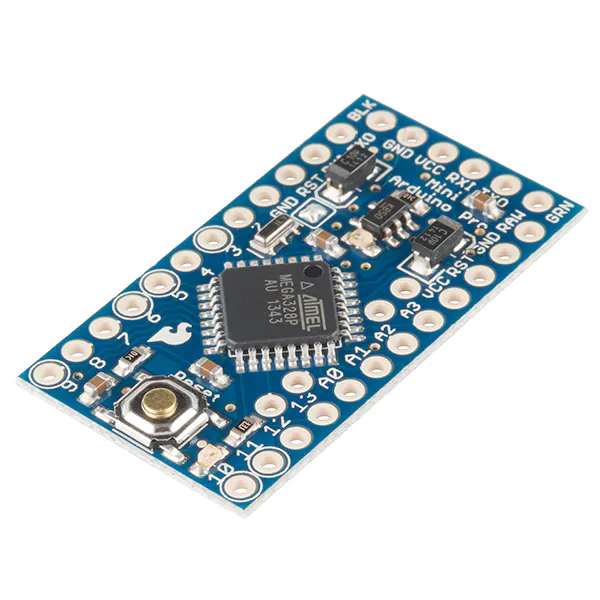SparkFun Arduino Pro Mini 328 - 5V/16MHz
×1
 LMD18200t
×1
 PCB
×1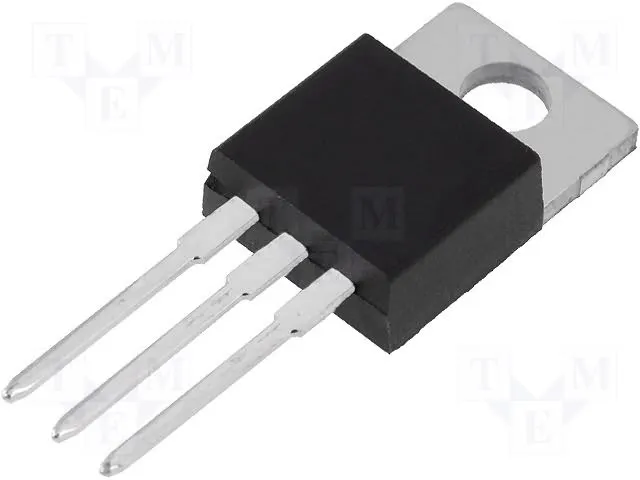Linear Regulator (7805)
×1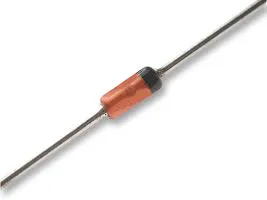3.6V 0.5W Zener Diode
×1HC-06 Bluetooth Module
×1
 0.1 ohm 2 watt resistor
×1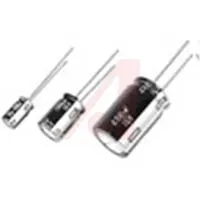Capacitor 220 µF 16 volt
×1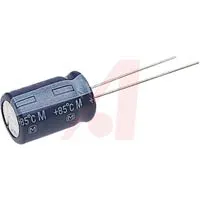Capacitor 10 µF 16 volt
×1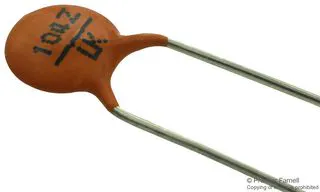Ceramic Disc Capacitor, 0.1 µF
×3Resistor 10k ohm
×2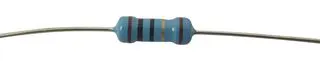Through Hole Resistor, 4.7 kohm
×1
 screw terminal 2 position 3.5 mm centres, 1.1 mm pin dia
×1
 screw terminal 6 position 2.54 mm centres
×1
 4 pin 2.54 mm socket
×1
 12 pin 2.54 mm socket
×2

## Apps and online services

 LocoMotive This is the free version of the App

A very simple electronic circuit provide the DCC signal and power for the track, however the App does the real work ! The computer on your phone is utilised to the full by constructing the codes required to form each packet of instructions, thus simplifying the job of the Arduino controller !

Update 16 August 2019: Release of new improved App - with facility to Read / Write CV 1 to 255
This App version costs £5 plus tax and available on the Google Play Store.

Update 18 May 2019: Release of new improved App - up to 28 functions with editable titles, visibility and momentary options. Control up to 4 locos at a timewith speed bars on the screen. This App version costs £3 plus tax and available on the Google Play Store.

Update 17 Feb 2019: You may have to modify the HC-06 Bluetooth module to extend its range of reception.I added a solid copper wire (from mains earth wire) 31.2 mm in length (1/4 of wave length for 2.4 GHz). See attached photo in Step 2.

Update 29 Jan 2019: Addition of momentary option on F2 and F3 for horn sounds Preset max speed for each locoThis is the most efficient DCC command station possible to build. No other system can do so much with so few components.An easy to assemble fully functioning DCC Command station with PCB available on eBay plus requiring only a few parts.The App 'LocoMotive' is designed to operate on Android phones running version 4.1 and upwards.No need to purchase any propriety systems, this is a stand alone unit operated by a free App called 'LocoMotive'Works with NMRA compliant decoders. This system compiles the operating DCC packets within the App which are sent to the Arduino interface to combine with the DCC clock signals. Purchase this PCB on eBay.Get free App LocoMotive on Google Play Store.Features include:

• Control of 1 to 20 locos
• Ideal for small to medium size layouts
• 2 Amps load drives up to 16 of OO/HO locomotives using H-bridge specified
• Short circuit protected
• Automatic over current cut-out, adjustable in Arduino code
• Lights and direction
• Functions 1 to 8
• Edit function names, visibility and momentary action
• Turnout / points / accessories operates up to 8 pairs of outputs
• Edit turnout names
• Custom naming of your locos and set max speed for each
• Programming the CV1 loco address
• Read / write CV 1 to 255
• Choose a DC power source to suit scale used (Z/N/OO/HO/O/G) 12v to 20v

Further to previous work on DCC wireless systems, I have developed a Bluetooth Command Station linked to a receiver Arduino based circuit with a HC-06 BT module and a LMD18200 H-bridge Motor driver delivering 2 Amps.

The overall cost of parts is around £20 with parts purchased from eBay.

### Step 1: Arduino Sketch

The full sketch is attached. This portion is the loop and data receiver code. The Android App sends a complete packet to the receiver and is decoded here into an array of data. Depending on whether 3 or 4 bytes are received the code will generate the correct DCC packet format for the train track.

void loop() {if(bluetooth.available() > 0); {inString = bluetooth.readStringUntil('\n');}if (inString.substring(0, 1)== "D") {string();}delay(20);}

The string() void then splits out the data from the text string received and places each instruction into itsappropriate position in the DCC packet.

For example:

A received text string of 'DD, 3, 63, 12, 48, ' translates into a 4 byte Speed packet of 8 bits each :

DD = message IDByte:    Decimal:     Binary: [0000 0011] [0001 1111] [0000 1100] [0001 1000] Action: [Loco 3] [speed steps] [ loco speed] [ XOR data check]

A received text string of 'DD, 1, 129, 128, ' translates into a 3 byte Function packet of 8 bits each :Decimal:    Binary: [0000 0001] [1000 0001] [1000 0000] Action: [Loco 1] [ F1 on] [XOR data check]

The LMD requires the DCC signal on the DIR pin and the PWM pin held HIGH.

### Step 2: Fritzing Circuit Diagram

The Arduino Pro Mini receives the Bluetooth data via the HC-06 module. The Arduino generates the NMRA standard clock cycles of data in packet format. The received data interrupts the sequence when new data is received and the DCC packet is updated to send instructions to the train tracks.

List of parts required:

PCB available on eBay.

1 off Arduino Pro Mini Atmega328P 5V/16M eBay

1 off LMD18200T H-bridge eBay

1 off HC-06 Bluetooth module eBay

1 off 0.1 ohm 2W Metal Film Resistor 0.1R 2 Watt (11.5 mm x 4.5 mm) eBay

3 off Capacitor 0.1uf eBay

2 off 10kΩ Resistor eBay

1 off Capacitor 10uf 25v; eBay

1 off Capacitor 220uf 16v; eBay

1 off Phoenix Contact MKDS 1/ 2-3, 5 2 Way Screw PCB Terminal Block 13.5A 200V 3.5mm eBay

1 off 4.7kΩ Resistor eBay

1 off L7805 CV Positive Voltage Regulator IC eBay

1 off 4 pin Female Header Edge Pins Strip 0.1" 2.54mm eBay

2 off 12 pin Female Header Edge Pins Strip 0.1" 2.54mm eBay

1 off 6 pin 2.54mm PCB Universal Screw Terminal Block eBay

1 off Zener Diode 3.6V; 0.5W 1N4732A eBay

Wire

Power `supply: For OO/HO layouts use a 14v DC power supply (e.g. laptop charger unit from eBay). For example this delivers up to 2.5 Amps. Check the size of panel mounted socket required to fit the DC jack plug on the power supply chosen for this project. https://www.ebay.co.uk/itm/Samsung-AC-DC-Adapter-...

To extend the range of the HC-06 Bt module, solder a wire onto the end of the antenna on the module. I used a solid copper wire (from mains earth wire) 31.2 mm in length (1/4 of wave length for 2.4 GHz). See photo above.Please note - the HC-06 connections are Rx (on PCB) to Rx (on module) and Tx to Tx. Please note, the antenna wire should be covered in sleeving to avoid accidental shorting with other components.

For the use of a LMD18200 h-bridge module (as displayed above this is in module form with heatsink etc), I have added current sense components to provide protection from short circuit or overload issues on the train track, The updated circuit diagram and Arduino code now includes the current sense function.

The code limits the max current to 2 Amps when reached, the LMD18200 h-bridge is shut down by applying a HIGH signal from D13 on the Arduino to the 'Brake' input on the h-bridge.

The use of a 0.1 ohm current sense resistor gives an analog input range of 0 to 200 mv on Arduino sensor pin A0 for up to 2 Amps load. For approx 5mv per analog step input (1023 total steps or divisions) gives 0 to 40 divisions or 2 Amps / 40 = 50mA per division. The measured current precision is 50 mA however we are protecting the h-bridge when 2 Amps is reached. The LMD18200 also has in-built over temperature protection as a back up.if(C >2000){ // 2 amps

Serial.println("Over Current"); digitalWrite(13, HIGH); }

The value of the current drawn is sent to the Android App via Bluetooth every second.void send_c(){t = millis() - lastmillis; if (t >= interval){ bluetooth.write(sensorValue); bluetooth.write('c'); lastmillis = millis(); } }

### Step 3: Android App

Instructions are attached for the full version App.

## Code

##### locomotive_dcc_sep19.inoArduino
Arduino Code
```// 1 Sep 2019
// sketch for use with Android App 'Locomotive DCC'using LMD18200 h-bridge

#include <SoftwareSerial.h>

SoftwareSerial bluetooth(8,9);  // RX TX
int C;
int sensorValue;
long t = 0;
long lastmillis = 0;
long interval = 1000;
String inString;
int a;
int preamable_type = 0;
float CV_VAL;
float cv_val;
float cv_val0;
float cv_val1;
float cv_val2;
float cv_val3;
float cv_val4;
float cv_val5;
float cv_val6;
float cv_val7;
int test_num;
int cv_write_val;
int Cs;
boolean cv_logic;
boolean ok;
int num;

// use digital pins 6 and 5 for DCC out

//Timer frequency is 2MHz for ( /8 prescale from 16MHz )
#define TIMER_SHORT 0x8D  // 58usec pulse length
#define TIMER_LONG  0x1B  // 116usec pulse length

unsigned char last_timer=TIMER_SHORT;  // store last timer value

unsigned char flag=0;  // used for short or long pulse
unsigned char every_second_isr = 0;  // pulse up or down

// definitions for state machine
#define PREAMBLE 0
#define SEPERATOR 1
#define SENDBYTE  2

unsigned char state= PREAMBLE;
unsigned char preamble_count = 16;
unsigned char outbyte = 0;
unsigned char cbit = 0x80;

// variables

unsigned char  xdata = 0, data = 0, data_f = 0,data_f1 = 0,data_f2 = 0;

int locoAdr = 9;   // this is the default address of the loco

// buffer for command
struct Message {
unsigned char data;
unsigned char len;
} ;

#define MAXMSG 3
// for the time being, use only 2 messages - the idle msg, the loco Speed msg, function msg

struct Message msg[MAXMSG] = {
{ { 0xFF,0, 0xFF, 0, 0, 0, 0}, 3},   // idle msg
{ { locoAdr, 0, 0,  0, 0, 0, 0}, 3}   // locoMsg with 128 speed steps 0x3f
};               // loco msg must be filled later with speed and XOR data byte

int msgIndex=0;
int byteIndex=0;

//Setup Timer2.
//Configures the 8-Bit Timer2 to generate an interrupt at the specified frequency.

void SetupTimer2(){
//Timer2 Settings: Timer Prescaler /8, mode 0
//Timmer clock = 16MHz/8 = 2MHz oder 0,5usec
TCCR2A = 0;
TCCR2B = 0<<CS22 | 1<<CS21 | 0<<CS20;

//Timer2 Overflow Interrupt Enable
TIMSK2 = 1<<TOIE2;

//load the timer for its first cycle
TCNT2=TIMER_SHORT;
}
//Timer2 overflow interrupt vector handler
ISR(TIMER2_OVF_vect) {
//Capture the current timer value TCTN2. This is how much error we have
//due to interrupt latency and the work in this function
//Reload the timer and correct for latency.
unsigned char latency;
// for every second interupt just toggle signal
if (every_second_isr)  {
PORTD = B01100000;  //use this instead of digitalWrite(6,1); digitalWrite(5,1);for LMD18200 ---  5 to 'DIR' DCC signal and 6 to 'PWM' held HIGH
//digitalWrite(6,1); digitalWrite(5,1);
every_second_isr = 0;
// set timer to last value
latency=TCNT2;
TCNT2=latency+last_timer;
}  else  {  // != every second interrupt, advance bit or state
PORTD = B01000000;  //for LMD18200 digitalWrite(6, 1); digitalWrite(5, 0)
//digitalWrite(6, 1); digitalWrite(5, 0);
every_second_isr = 1;
switch(state)  {
case PREAMBLE:
flag=1; // short pulse
preamble_count--;
if (preamble_count == 0)  {  // advance to next state
state = SEPERATOR;
// get next message
msgIndex++;
if (msgIndex >= MAXMSG)  {  msgIndex = 0; }
byteIndex = 0; //start msg with byte 0
}
break;
case SEPERATOR:
flag=0; // long pulse
// then advance to next state
state = SENDBYTE;
// goto next byte ...
cbit = 0x80;  // send this bit next time first
outbyte = msg[msgIndex].data[byteIndex];
break;
case SENDBYTE:
if (outbyte & cbit)  {
flag = 1;  // send short pulse
}  else  {
flag = 0;  // send long pulse
}
cbit = cbit >> 1;
if (cbit == 0)  {  // last bit sent, is there a next byte?
byteIndex++;
if (byteIndex >= msg[msgIndex].len)  {
state = PREAMBLE;
if (preamable_type == 0){
preamble_count = 16;    // normal preamble length of 16 '1's
}
if (preamable_type == 1){
preamble_count = 24;    // preamble of 24 '1's for CV1 write
}
}  else  {
// send separtor and advance to next byte
state = SEPERATOR ;
}
}
break;
}
if (flag)  {  // if data==1 then short pulse
latency=TCNT2;
TCNT2=latency+TIMER_SHORT;
last_timer=TIMER_SHORT;
}  else  {   // long pulse
latency=TCNT2;
TCNT2=latency+TIMER_LONG;
last_timer=TIMER_LONG;
}
}
}

void setup(){
Serial.begin(115200);
analogReference(INTERNAL);
pinMode(13, OUTPUT);

bluetooth.begin(9600);
delay(500); // wait for bluetooth module to start
Serial.println("Bluetooth Started");
bluetooth.println("Bluetooth Started");

DDRD = B01100000;   //  register D5 for digital pin 5, D6 for digital pin 6
//Start the timer
SetupTimer2();
t = millis();
lastmillis = millis();
digitalWrite(6, LOW);
digitalWrite(13, HIGH); // turn bridge o/p off

int get_cv_val = cv_calc(test_num);
}

void current(){
int i;
int value = 0;
for (i = 0; i < numReadings; i++){
delay(1);
}

// Convert the analog reading (which goes from 0 - 1023) to a voltage (0 - 1.1V) = 1.08mv per division
// 0.1 ohm resistor on current sense gives 200mv at 2 Amps or, 100mv per Amp
// 1.08 mv per div for Internal Ref of 1.1v  : 100/1.08 = 92.6 divisions per 1000mA or 1 div = 10.8mA
C = 10.8 * sensorValue ;  // mA

if(C >2000){  // 2 amps
Serial.println("Over Current");
bluetooth.println("Short!");
digitalWrite(6, LOW);  // logic control of DCC signal to low (off)
digitalWrite(13, HIGH); // to brake, shorts out h-bridge o/p
bluetooth.println(C);
}
}

void loop() {

if(bluetooth.available() == true);
{
Serial.println(inString);
}
if (inString.substring(0,1) == "D"){
string(); }
// incoming DCC packet
if (inString.substring(0,1) == "C"){
digitalWrite(6, HIGH); digitalWrite(13, LOW); }
// reset after over current, logic control of DCC signal on,brake off
if (inString.substring(0,1) == "G"){
digitalWrite(6, HIGH); digitalWrite(13, LOW);
current();
bluetooth.println(C);}
// request for load current value
if (inString.substring(0,1) == "A"){
digitalWrite(6, HIGH); digitalWrite(13, LOW);
get_cv_data();
cv1_prog();
digitalWrite(6, LOW); digitalWrite(13, HIGH);}
if (inString.substring(0,3) == "get"){
cv_val = 0;
digitalWrite(6, HIGH); digitalWrite(13, LOW);
get_cv_num();

digitalWrite(6, LOW); digitalWrite(13, HIGH);
}
cv_write_val = 0;
digitalWrite(6, HIGH); digitalWrite(13, LOW);
get_cv_new_val();
repeat_cv_write();
digitalWrite(6, LOW); digitalWrite(13, HIGH);
}
if (inString.substring(0,1) == "V"){
delay(50);bluetooth.print("CV1 updated");
// cv1 write
if (inString.substring(0,1) == "E"){
digitalWrite(6, LOW);  // logic control of DCC signal to low (off)
digitalWrite(13, HIGH); // to brake, shorts out h-bridge o/p
}   // e-stop
inString = "";
}

void get_cv_new_val(){
Serial.println(inString);
String cv_w ="";
int x = inString.indexOf("new,") + 4;
cv_w = inString.substring(x,x+3);
Serial.println(cv_w);
cv_write_val = cv_w.toInt();
Serial.print("cv_write_val: ");Serial.println(cv_write_val);

int z = inString.indexOf("addr,") + 5;
}

void get_cv_num(){
cv_write_val = 0;
Serial.println(inString);
String temp ="";
int x = inString.indexOf(",") + 1;
temp = inString.substring(x,8);
Serial.println(temp);
}

void get_cv_data(){
unsigned long z = inString.length();
int y = 0;
int count = 0;
String inChar;
String temp ="";
for (int i = 0; i<=z; i++){
inChar = inString.substring(i,i+1);
if (inChar == ",") {count++;}
if (inChar != "," && inChar != "A") {temp += inChar;}
if (inChar == ",") {Address = (temp.toInt());y = y +1;temp = "";}
}
amend_len3(msg);
assemble_3_byte();
Serial.println(inString);
}

void string(){
unsigned long z = inString.length();
int y = 0;
for (int i = 0; i<=5; i++){
a[i] = 0;
}
int count = 0;
String inChar;
String temp ="";
for (int i = 0; i<=z; i++){
inChar = inString.substring(i,i+1);
if (inChar == ",") {
count++;
}
if (inChar != "," && inChar != "D") {
temp += inChar;
}
if (inChar == ",") {
a[y] = (temp.toInt());
y = y +1;
temp = "";
}
}

if (count == 3){
//Serial.println(inString);
//print_data();
amend_len3(msg);
assemble_3_byte();
}
if (count == 4){
//Serial.println(inString);
//print_data();
amend_len4(msg);
assemble_4_byte();
}
//Serial.println(inString);
//Serial.println(a);
// Serial.println(a);
//Serial.println(a);
//Serial.println(a);
//print_data();
}

void amend_len4 (struct Message & x)
{
x.len = 4;
//Serial.println(x.len);
}

void assemble_4_byte() {
preamable_type = 0;  // 16 x '1's
noInterrupts();
msg.data = B11111111;
msg.data = B00000000;
msg.data = B11111111;
msg.data = a;
msg.data = a;
msg.data = a;
msg.data = ((a ^ a)^ a);
interrupts();
}

void amend_len3 (struct Message & x)
{
x.len = 3;
//Serial.println(x.len);
}

void assemble_3_byte() {
preamable_type = 0;  // 16 x '1's
noInterrupts();
msg.data = B11111111;
msg.data = B00000000;
msg.data = B11111111;
msg.data = a;
msg.data = a;
msg.data = (a ^ a);
msg.data = 0;
interrupts();

}

void print_data(){
Serial.print(msg.data, DEC);
Serial.print(",");
Serial.print(msg.data, DEC);
Serial.print(",");
Serial.print(msg.data, DEC);
Serial.print(",");
Serial.print(msg.data, DEC);
Serial.println(",");
}

void cv_current(){
for (int i = 1 ; i<=10 ; i++){
C = 10.8 * sensorValue ;  // mA
if (C >= Cs){
cv_logic = true;
}
delayMicroseconds(500);
Serial.print("C = ");Serial.println(C);
}
}

cv_val = 0;
delay(200);
current();
if(bluetooth.available() == true);{
}
Cs = C + 50;
CV_VAL = 99;
for (int f = 1 ; f<=10 ; f++){
if (CV_VAL == 99){
cv_val0 = cv_calc(0);
delay(10);
} }
CV_VAL = 99;
for (int f = 1 ; f<=10 ; f++){
if (CV_VAL == 99){
cv_val1 = cv_calc(1);
delay(10);
} }

CV_VAL = 99;
for (int f = 1 ; f<=10 ; f++){
if (CV_VAL == 99){
cv_val2 = cv_calc(2);
delay(10);
} }

CV_VAL = 99;
for (int f = 1 ; f<=10 ; f++){
if (CV_VAL == 99){
cv_val3 = cv_calc(3);
delay(10);
} }

CV_VAL = 99;
for (int f = 1 ; f<=10 ; f++){
if (CV_VAL == 99){
cv_val4 = cv_calc(4);
delay(10);
} }

CV_VAL = 99;
for (int f = 1 ; f<=10 ; f++){
if (CV_VAL == 99){
cv_val5 = cv_calc(5);
delay(10);
} }

CV_VAL = 99;
for (int f = 1 ; f<=10 ; f++){
if (CV_VAL == 99){
cv_val6 = cv_calc(6);
delay(10);
} }

CV_VAL = 99;
for (int f = 1 ; f<=10 ; f++){
if (CV_VAL == 99){
cv_val7 = cv_calc(7);
delay(10);
} }

Serial.print("cv_val0 ");Serial.println(cv_val0);
Serial.print("cv_val1 ");Serial.println(cv_val1);
Serial.print("cv_val2 ");Serial.println(cv_val2);
Serial.print("cv_val3 ");Serial.println(cv_val3);
Serial.print("cv_val4 ");Serial.println(cv_val4);
Serial.print("cv_val5 ");Serial.println(cv_val5);
Serial.print("cv_val6 ");Serial.println(cv_val6);
Serial.print("cv_val7 ");Serial.println(cv_val7);
int cv_val_total = cv_val0+cv_val1+cv_val2+cv_val3+cv_val4+cv_val5+cv_val6+cv_val7;
cv_val = 0;
if (cv_val0 == 1){
cv_val = cv_val + 1;
}
if (cv_val1 == 1){
cv_val = cv_val + 2;
}
if (cv_val2 == 1){
cv_val = cv_val + 4;
}
if (cv_val3 == 1){
cv_val = cv_val + 8;
}
if (cv_val4 == 1){
cv_val = cv_val + 16;
}
if (cv_val5 == 1){
cv_val = cv_val + 32;
}
if (cv_val6 == 1){
cv_val = cv_val + 64;
}
if (cv_val7 == 1){
cv_val = cv_val + 128;
}

if(bluetooth.available() == true);{
if (cv_val_total > 9){
}
if (cv_val_total < 9){
bluetooth.println(int(cv_val + 0.5));
}
}
Serial.print("cv_val ");Serial.println(int(cv_val + 0.5));
}

void cv1_prog(){
for (int i = 0 ; i<=3 ; i++){
reset_packet();
delay(5);
}
for (int i = 0 ; i<=5 ; i++){
page_preset_packet();
delay(5);
}

for (int i = 0 ; i<=9 ; i++){
reset_packet();
delay(5);
}
for (int i = 0 ; i<=5 ; i++){
cv1_write_packet();
delay(5);
}
current();
for (int i = 0 ; i<=10 ; i++){
cv1_write_packet();
delay(5);
}
}

void reset_packet() {
noInterrupts();
preamable_type = 0;
msg.data = B11111111;
msg.data = B11111111;
msg.data = B11111111;
msg.data = B00000000;
msg.data = B00000000;
msg.data = B00000000;
interrupts();
}
void page_preset_packet() {
noInterrupts();
preamable_type = 0; // 16 x '1's
msg.data = B11111111;
msg.data = B11111111;
msg.data = B11111111;
msg.data = B01111101;
msg.data = B00000001;
msg.data = B01111100;
interrupts();
}
void cv1_write_packet() {
preamable_type = 1;  // 24 x '1's
noInterrupts();
msg.data = B11111111;
msg.data = B11111111;
msg.data = B11111111;
msg.data = B01111000; // Address only mode
msg.data = (msg.data ^ msg.data);
interrupts();
}

void cv_verify1_packet() {
noInterrupts();
preamable_type = 1;  // 24 x '1's
msg.data = B11111111;
msg.data = B11111111;
msg.data = B11111111;
msg.data = B01111000; // bit manipulation mode
msg.data = B00000000 | (Address -1);
msg.data = B11101000 | num;
msg.data = (msg.data ^ msg.data) ^ msg.data;
interrupts();
}

void cv_verify0_packet() {
noInterrupts();
preamable_type = 1;  // 24 x '1's
msg.data = B11111111;
msg.data = B11111111;
msg.data = B11111111;
msg.data = B01111000; // bit manipulation mode
msg.data = B00000000 | (Address -1);
msg.data = B11100000 | num;
msg.data = (msg.data ^ msg.data) ^ msg.data;
interrupts();
}

void cv_write_packet() {
preamable_type = 1;  // 24 x '1's
noInterrupts();
msg.data = B11111111;
msg.data = B11111111;
msg.data = B11111111;
msg.data = B01111100; // write mode
msg.data = B00000000 | (Address -1);
msg.data = B00000000 | cv_write_val;
msg.data = (msg.data ^ msg.data) ^ msg.data;
interrupts();
}

void valid_packet(){
noInterrupts();
msg.data = B11111111;
msg.data = B00000000;
msg.data = B11111111;
preamable_type = 0; // 16 x '1's
msg.data = 0x80;   // locoMsg with group one instruction 0x80
msg.data = msg.data ^ msg.data;
msg.data = 0;
interrupts();
}

//CV write
void repeat_cv_write() {
current();
Cs = C + 50;
Serial.print("C = ");Serial.println(C);
Serial.print("Cs = ");Serial.println(Cs);
if(bluetooth.available() == true);{
bluetooth.print("writing..");
}

ok = false;
for (int f = 1 ; f<=10 ; f++){
if (ok == false){
cv_write();
delay(10);
}
}
}

void cv_write(){
delay(100);
amend_len3(msg);
assemble_3_byte();
delay(5);
for (int i = 1 ; i<=20 ; i++){
valid_packet();
delay(5);
}

for (int i = 1 ; i<=6 ; i++){
reset_packet();
delay(5);
}
cv_logic = false;
amend_len4(msg);
assemble_4_byte();
for (int i = 1 ; i<=10 ; i++){
cv_write_packet();
cv_current();
Serial.print("C= ");Serial.println(C);
if (cv_logic){
ok = true;
cv_logic = false;
i = 11;
amend_len3(msg);
assemble_3_byte();
for (int i = 1 ; i<=10 ; i++){
reset_packet();
delay(5);
}
if(bluetooth.available() == true);{
bluetooth.print("write = ");
bluetooth.println(cv_write_val);
Serial.print("cv_write_val ");Serial.println(cv_write_val);
}
}
}
if(bluetooth.available() == true);{
if (ok == false){
bluetooth.print("write = error");
}
}

}

int cv_calc(int test_num) {

amend_len3(msg);
assemble_3_byte();
delay(5);
for (int i = 1 ; i<=20 ; i++){
valid_packet();
delay(5);
}
num = test_num;
amend_len3(msg);
assemble_3_byte();
for (int k = 1 ; k<=4 ; k++){
reset_packet();
delay(5);
}
amend_len4(msg);
assemble_4_byte();
cv_logic = false;
delay(2);

for (int m = 1 ; m<=5 ; m++){
cv_verify1_packet();
cv_current();
if (cv_logic){
cv_logic = false;
CV_VAL =  1;
Serial.print("test_num = ");Serial.println(test_num);
Serial.println(CV_VAL);
preamable_type = 0;
amend_len3(msg);
assemble_3_byte();
delay(5);
for (int j = 1 ; j<=3 ; j++){
reset_packet();
delay(5);
}
}
}

Serial.println("test for 0 ");
amend_len3(msg);
assemble_3_byte();
for (int k = 1 ; k<=4 ; k++){
reset_packet();
delay(5);
}
amend_len4(msg);
assemble_4_byte();
cv_logic = false;
delay(2);
for (int m = 1 ; m<=5 ; m++){
cv_verify0_packet();
cv_current();

if (cv_logic){
cv_logic = false;
CV_VAL = 0;
Serial.print("test_num = ");Serial.println(test_num);
Serial.println(CV_VAL);
preamable_type = 0;
amend_len3(msg);
assemble_3_byte();
delay(5);
for (int j = 1 ; j<=3 ; j++){
reset_packet();
delay(5);
}
}
}
Serial.println(CV_VAL);
return CV_VAL;
}
```

## Custom parts and enclosures

Complete circuit within enclosure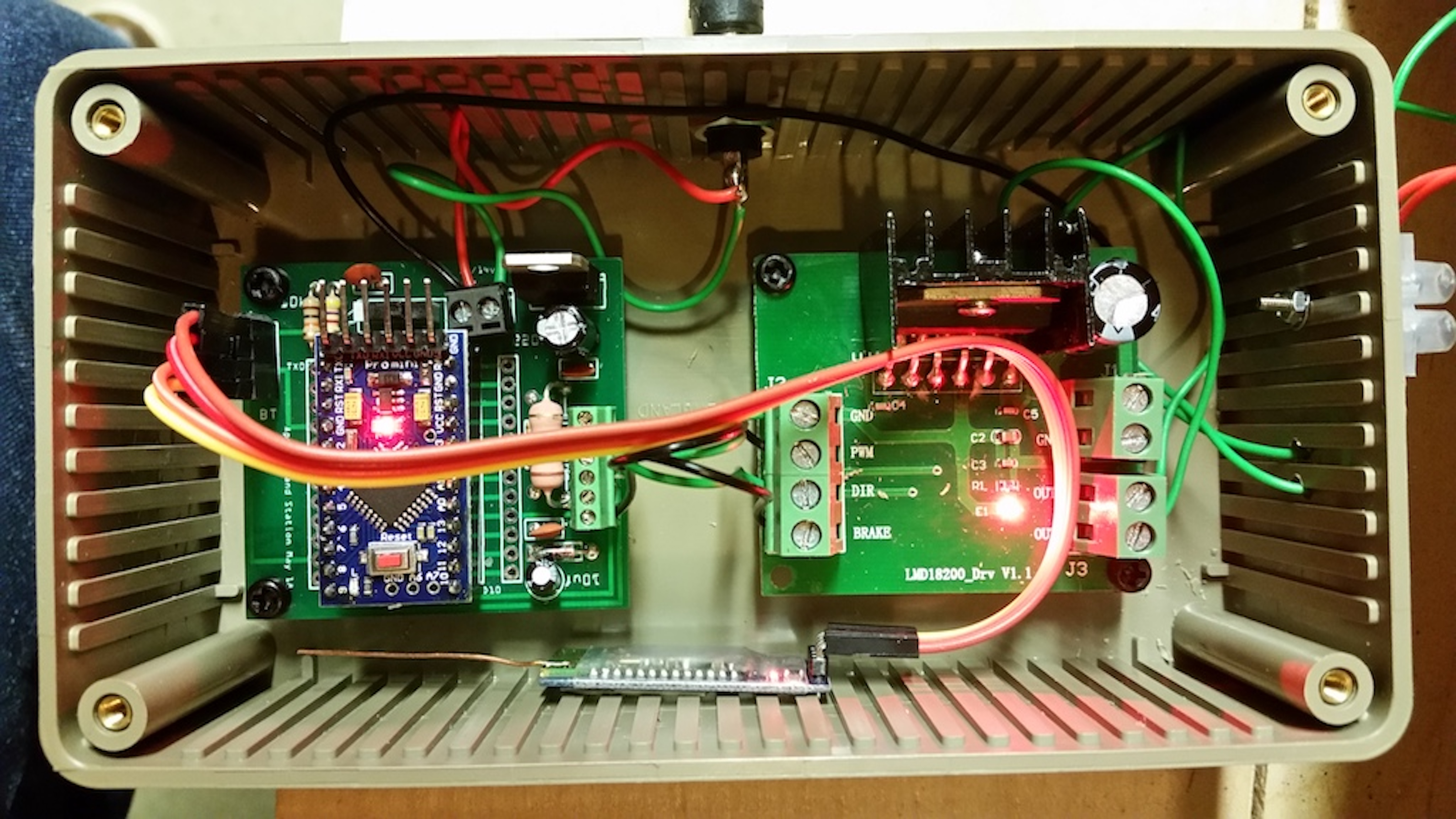## Schematics

Bluetooth receiver / H-Bridge amplifier circuit
bluetooth_android_dcc_lmd18200_S8NTVsI4LY.fzz
App instructions

April 30, 2019

#### Members who respect this project

See similar projects
you might like

#### Arduino Bluetooth RC Car

Project tutorial by ardumotive

• 3,622 views
• 14 respects

#### RC Car Hack With Android And Arduino

Project showcase by danionescu

• 13,717 views
• 15 respects

#### Create a People Counter Controlled by an Android App

Project tutorial by Kutluhan Aktar

• 6,865 views
• 8 respects

Project tutorial by Kutluhan Aktar

• 4,397 views
• 16 respects

#### Mobile Water Level Tracker

Project tutorial by Kutluhan Aktar

• 2,998 views